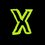# Inequalities: Intervals

Hello everyone! this is my first note in the Inequalities series. I'll be posting many notes and whacky questions in this series, so stay tuned and enjoy mathematics. PS : These notes will be of REAL help for IIT preparation as well.



$\mathbf{ INTERVALS }$ Set of numbers between any two real numbers ( $\mathbb{ R }$ ) is called an interval.

For example: Consider a variable $x$ whose possible values lies strictly between $1$ and $10$, including $10$. Thus, we can say that: $1< x \leq 10$

[ NOTE1: Since $x \neq 1$, we write $1< x \leq 10$ and not $1\leq x \leq 10$ ]

Alternatively, this can be represented in interval form as:$x \in (1,10]$



In general, for $x , a , b \in \mathbb{R} | a < b$

\begin{aligned} a < x < b & \Rightarrow x \in (a,b) \\ a \leq x < b & \Rightarrow x \in [a,b) \\ a < x \leq b & \Rightarrow x \in (a,b] \\ a \leq x \leq b & \Rightarrow x \in [a,b] \end{aligned}



This interval form can be represented on the number line as follows :[ NOTE2: We never use a closed interval for $\pm \infty$. In other words, we never equate the variable to infinity. For example: Set of all real numbers can be expressed as $( - \infty , \infty)$ ]



$\mathbf{ PRACTICE }$

1. If the roots of the following equation belong to the set $(a, \infty)$. Find the maximum possible integral value of a. $2x^2 - x -1 = 0$

2. Find the number of integers ( $\mathbb(Z)$ ) satisfying $\frac{-17}{4} < x < 11$

3. Frame your own interesting question and post it down below in the comments.



I sincerely thank each one of you for reading this note. I'll try my best to make myself more understandable in the next notes. Comment below your views , answers and share interesting questions related to it.

Stay tuned for more notes and questions. You can even post your matter down here in the comments to make this topic more interesting. (I've reserved the advanced topics and properties for upcoming notes.)

Use #PSQuest to see more from me.

Complete set here : Inequalities

Like and Share :)Note by Priyansh Sangule
6 years, 11 months ago

This discussion board is a place to discuss our Daily Challenges and the math and science related to those challenges. Explanations are more than just a solution — they should explain the steps and thinking strategies that you used to obtain the solution. Comments should further the discussion of math and science.

When posting on Brilliant:

• Use the emojis to react to an explanation, whether you're congratulating a job well done , or just really confused .
• Ask specific questions about the challenge or the steps in somebody's explanation. Well-posed questions can add a lot to the discussion, but posting "I don't understand!" doesn't help anyone.
• Try to contribute something new to the discussion, whether it is an extension, generalization or other idea related to the challenge.
• Stay on topic — we're all here to learn more about math and science, not to hear about your favorite get-rich-quick scheme or current world events.

MarkdownAppears as
*italics* or _italics_ italics
**bold** or __bold__ bold
- bulleted- list
• bulleted
• list
1. numbered2. list
1. numbered
2. list
Note: you must add a full line of space before and after lists for them to show up correctly
paragraph 1paragraph 2

paragraph 1

paragraph 2

[example link](https://brilliant.org)example link
> This is a quote
This is a quote
    # I indented these lines
# 4 spaces, and now they show
# up as a code block.

print "hello world"
# I indented these lines
# 4 spaces, and now they show
# up as a code block.

print "hello world"
MathAppears as
Remember to wrap math in $$ ... $$ or $ ... $ to ensure proper formatting.
2 \times 3 $2 \times 3$
2^{34} $2^{34}$
a_{i-1} $a_{i-1}$
\frac{2}{3} $\frac{2}{3}$
\sqrt{2} $\sqrt{2}$
\sum_{i=1}^3 $\sum_{i=1}^3$
\sin \theta $\sin \theta$
\boxed{123} $\boxed{123}$

## Comments

Sort by:

Top Newest

(FUVEST 2012) Determine for which real intervals of $x$ the following inequality holds. $|x^2-10x+21| \leq |3x-15|$

- 6 years, 11 months ago

Log in to reply

Whoa @Guilherme Dela Corte isn't it too early for the newbies ?

Anyways thanks for posting. I'll include this problem in my future notes :)

- 6 years, 11 months ago

Log in to reply

×

Problem Loading...

Note Loading...

Set Loading...